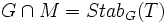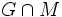# Set-stabilizer in a nilpotent group problem

This article describes a group-finding problem, that is, a problem where we have to explicitly find a generating set of a group that is specified through certain conditions

This is a variation of set-stabilizer problem|Find other variations of set-stabilizer problem |

## Description

### Given data

A nilpotent group$G$ acts faithfully on a set$S$, and it is described by a generating set$A$. We are given a subset$T \subseteq S$.

### Goal

We need to find the set-stabilizer of$T$ in$G$, that is, the set of elements of$G$ that stabilize$T$.

## Solution

### Outline

Since$G$ is nilpotent,$G$ is the direct product of its Sylow subgroups. Further, the stabilizer of$T$ in$G$, being a subgroup of$G$, is also a direct product of its Sylow subgroups, hence it suffices to find the Sylow subgroups of the stabilizer. Further, the Sylow subgroups of the stabilizer are the same as the stabilizers in the Sylow subgroups.

That is, if$p$ is a prime and$P$ is a$p$-Sylow subgroup of$G$ then$Stab_P(T)$ is the$p$-Sylow subgroup of$Stab_G(T)$.

We thus need to do the following:

1. Find all the Sylow subgroups of$G$
2. Find the set-stabilizer in each Sylow subgroup
3. Take the direct product of those (or simply, take the union of the generating sets)

### Finding all the Sylow subgroups

This is easily achieved by looking at the original generating set and projecting onto the Sylow subgroups.

### Finding the set stabilizer in each Sylow subgroup

1. Construct a structure forest for$G$. Such a structure forest must be a union of complete binary trees.
2. Now, pair together structure trees of the same size, to get a complete$p$-ary tree with depth 1 more. Keep doing this pairing procedure (like binary addition) till all the trees have different sizes
3. Thus, obtain trees of different sizes. The automorphism group of this new forest is a Sylow$p. Call this Sylow subgroup$P$.
4. For all the vertices in$T$, attach a long gadget (say a path) from these vertices, in such a way that the only permissible automorphisms of the new tree (after attaching these gadgets) are automorphisms that send vertices of$T$ to among themselves.
5. Having put these gadgets, compute the automorphism group of the forest along with these gadgets. This can easily be done as it is a special case of finding the automorphism group of a rooted forest. Call this automorphism group$M$.

We now have a situation where:

•$G$ sits inside a 2-Sylow subgroup$P$
• There exists a subgroup$M$ containing the whole of$Stab_G(T)$ that also sits inside$P$ and such that$G \cap M = Stab_G(T)$
• Since$P$ is a 2-group, both$G$ and$M$ are subnormal in$G$. Thus, computing$G \cap M$ reduces to the subnormalizing group intersection problem.

### Taking the direct product

Simply take the union of all generating sets.